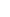12. FREQUENCY CHANGE IN DOPPLER SHIFTIn the electromagnetic interaction between reference systems that are in motion relative to each other, the frequency of a signal changes similarly to the way a signal's wavelength changes. However, there is an important detail here: Frequency Shift does not occur at the source where the signal is emitted, it is observed on the INCOMING signal on the side that receives the signal as a Relativity Effect.Frequency Shift is delineated in the figure above. The transmitter emits signals at f0 frequency. The station on the mountain receives this transmission at f0 frequency while the plane moving away receives it at f1 frequency and the plane coming at f2 frequency. Frequency Shift is described in the equations below.Frequency Shift Frames are moving away  from each otherFrames are approaching  each other(c+v) and (c-v) under the fraction bar in the equations are the speeds of signals going towards targets in motion relative to the reference system of the source of the signal. Here they sing the same song again: We are the speeds of the signals going to the planes from the transmitter. We are the speeds of the signals going to the planes from the transmitter.As there is the equation ofbetween the frequency and wavelength on the side of the receiver and transmitter, there isequation.The topic of Frequency Shift will appear in more detail again in the following chapters. I will just leave it aside for now.